• Amaelle

#### Amaelle

Homework Statement
Loook at the image
Relevant Equations
Power series.
Greetings
I have some problems finding the correct result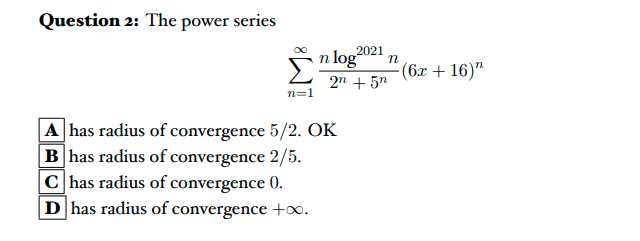My solution:
I puted Y=6x+16
so now will try to find the raduis of convergence of Y
so let's calculate the raduis criteria of convergence: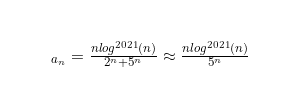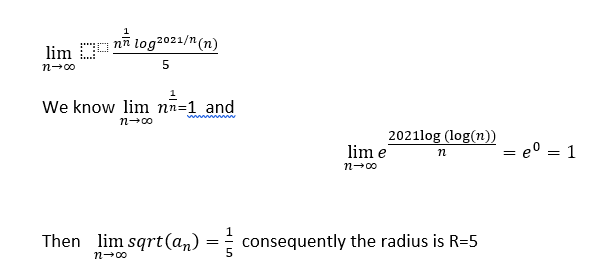• We know that Y=6x+16
• Conseqyently -21/6<=x<=-11/6 so the raduis must be 5/3. But this is not the solution!
Here is the solution of the book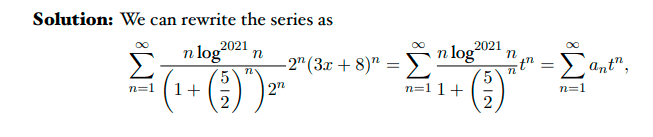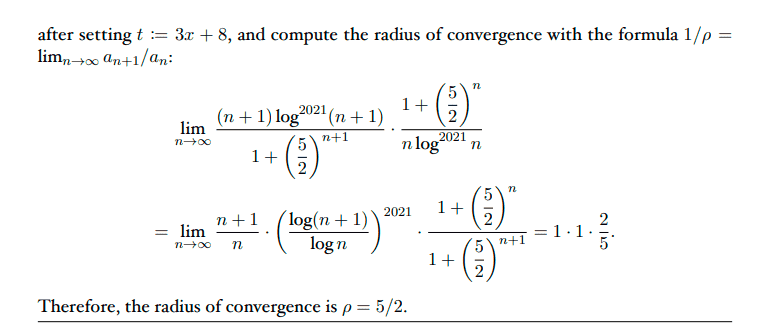I would like to know where is my mistake
thank you!

•Delta2
I am not sure about your notation $$\log^{2021}x$$. Is it
$$\log_{2021}x$$ or
$$(\log x)^{2021}$$?

•Amaelle
5 for 6x+16 and 5/2 for 3x+8 seem not different. What is the rule to define t for t^n series for convergence ?

•Delta2 and Amaelle
anuttarasammyak said:
I am not sure about your notation $$\log^{2021}x$$. Is it
$$\log_{2021}x$$ or
$$(\log x)^{2021}$$?
$$(\log x)^{2021}$$?

anuttarasammyak said:
5 for 6x+16 and 5/2 for 3x+8 seem not different. What is the rule to define t for t^n series for convergence ?

I realize that I have the same confusion, I thought we were looking for the domain where x is convergent?
I understand I got the same results implicitely but did the book go for that particular set up?
thanks a million!